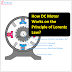Hey, in this article we are going to know how dc motor works on the principle Lorentz Law. Before that, we have to know what is Lorentz's law. The Lorentz law states that the electric force and magnetic force with combination create a new force and its direction can be stated by fleming's left-hand rule. Now if we assume that the electric field is E and the magnetic field is B. Now a particle of charge q moving with a velocity v in a magnetic field B and in an electric field E, so it will experience a force,

F = qE + qv x V

This generated force is known as Lorentz Force. It is also known as the electromagnetic force.

So you may understand the Lorentz Law simply states that if we placed a currying carrying conductor in a magnetic field a force will be created due to the combination or interaction of electrical force and the magnetic force. Now if any one of them is subjected to movable, then it will move. For example, if the current-carrying conductor is left as a movable it will move due to the Lorentz force.

Now come to the question, how the dc motor works on the principle of Lorentz law. We know that the DC motor has main two parts - 1. Stator Part and 2. Rotor Part

The stator part has field windings that will create a magnetic field when electrical power is supplied to it. For, the PMDC motor a permanent magnet is used instead of magnetic coils. The rotor part is consists of an armature coil, commutator, etc. So during the operation, when electrical supply is applied to the armature winding, the rotor or armature winding starts rotating.

For a separately excited DC Motor, the stator coils are divided according to the pole such as the north pole and the south pole. Basically, this is done by changing the direction of the wounding of the coil. There are so many parts in the stator of the motor such as pole face, pole shoe. There a laminated core is used where the coil is wound. This is because of reducing iron loss. According to the connection of the field coil, there are different types of DC Motor such as DC Shunt Motor, DC Series Motor, and DC Compound Motor. In the DC shunt motor, the field coil and armature coils are connected in parallel, whereas in the DC Series motor the field coil and armature coil is connected in series. In the compound motor, the series and parallel both of the combinations are used.

In a DC motor, the commutator plays a very important role. It converts the direct into alternating current. Generally, we provide the DC current through the brush. From the brush, the current goes to the commutator, and the commutator supplies the current to the armature coil converting it into alternating form. So all the arrangements are used for the proper operation of the motor.

Basically, the Lorentz force law explains the relationship between the magnetic field, electric current, and force experienced by a conductor in a DC motor. This understanding allows engineers to design and optimize DC motors for efficient and reliable operation in various industries and applications. The Lorentz force law is utilized to explain the motion and operation of the motor.

How DC Motor Works on the Principle of Lorentz Law?Reviewed by Author on February 10, 2022 Rating: 5